一、栈

(01) 栈中数据是按照"后进先出（LIFO, Last In First Out）"方式进出栈的。

(02) 向栈中添加/删除数据时，只能从栈顶进行操作。

push -- 向栈中添加元素。

peek -- 返回栈顶元素。

pop -- 返回并删除栈顶元素的操作。

1.栈的示意图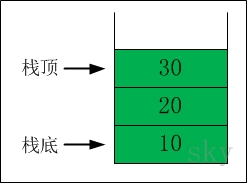2.出栈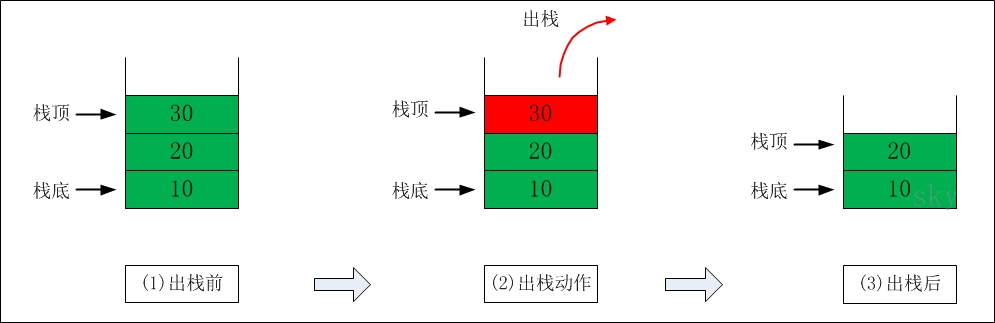3. 入栈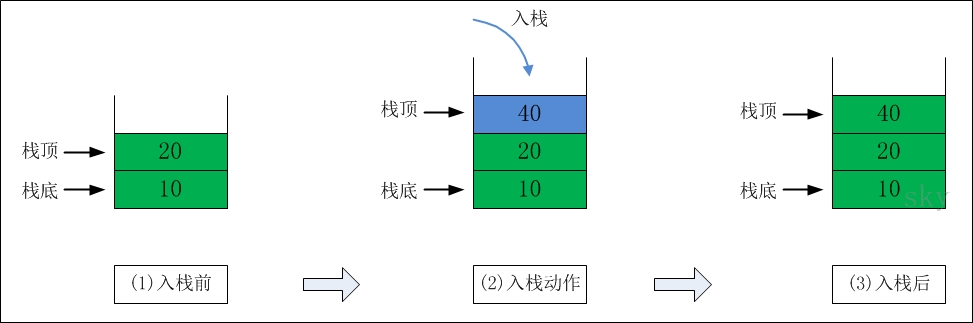4.栈的Java实现

JDK包中也提供了"栈"的实现，它就是集合框架中的Stack类。 本部分使用数组实现栈，能存储任意类型的数据。

/**
* Java : 数组实现的栈，能存储任意类型的数据
*
* @author skywang
* @date 2013/11/07
*/
import java.lang.reflect.Array;

public class GeneralArrayStack<T> {

private static final int DEFAULT_SIZE = 12;
private T[] mArray;
private int count;

public GeneralArrayStack(Class<T> type) {
this(type, DEFAULT_SIZE);
}

public GeneralArrayStack(Class<T> type, int size) {
// 不能直接使用mArray = new T[DEFAULT_SIZE];
mArray = (T[]) Array.newInstance(type, size);
count = 0;
}

// 将val添加到栈中
public void push(T val) {
mArray[count++] = val;
}

// 返回“栈顶元素值”
public T peek() {
return mArray[count-1];
}

// 返回“栈顶元素值”，并删除“栈顶元素”
public T pop() {
T ret = mArray[count-1];
count--;
return ret;
}

// 返回“栈”的大小
public int size() {
return count;
}

// 返回“栈”是否为空
public boolean isEmpty() {
return size()==0;
}

// 打印“栈”
public void PrintArrayStack() {
if (isEmpty()) {
System.out.printf("stack is Empty\n");
}

System.out.printf("stack size()=%d\n", size());

int i=size()-1;
while (i>=0) {
System.out.println(mArray[i]);
i--;
}
}
}

二、队列

1.队列的示意图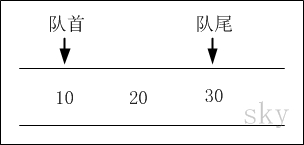2.出队列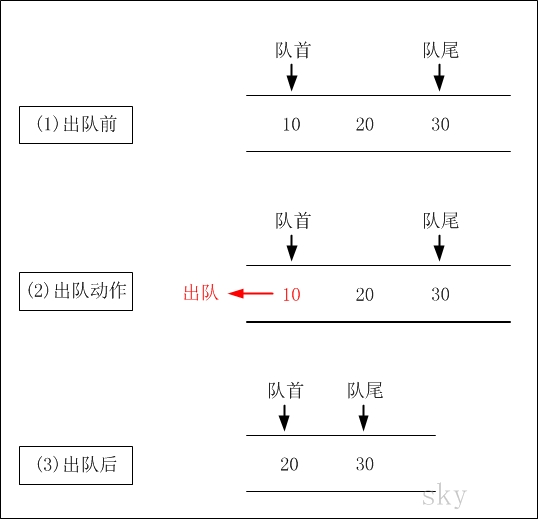3.入队列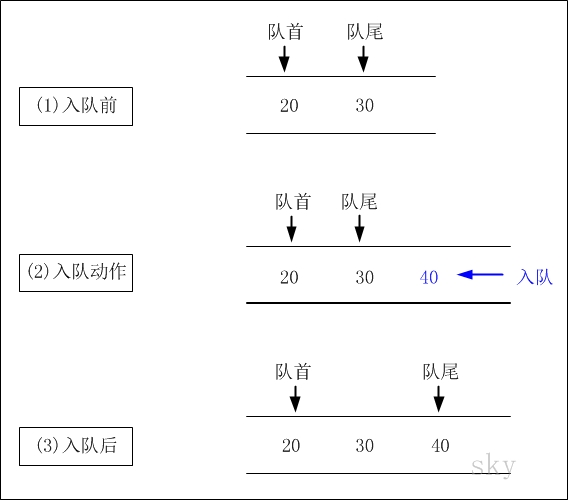4.队列的Java实现

/**
* Java : 数组实现“队列”，只能存储int数据。
*
* @author skywang
* @date 2013/11/07
*/
public class ArrayQueue {

private int[] mArray;
private int mCount;

public ArrayQueue(int sz) {
mArray = new int[sz];
mCount = 0;
}

// 将val添加到队列的末尾
public void add(int val) {
mArray[mCount++] = val;
}

// 返回“队列开头元素”
public int front() {
return mArray;
}

// 返回“队首元素值”，并删除“队首元素”
public int pop() {
int ret = mArray;
mCount--;
for (int i=1; i<=mCount; i++)
mArray[i-1] = mArray[i];
return ret;
}

// 返回“栈”的大小
public int size() {
return mCount;
}

// 返回“栈”是否为空
public boolean isEmpty() {
return size()==0;
}
}